# 1. static_cast

## 1.1 static_cast语法

static_cast< new_type >(expression)


C风格写法：

double scores = 96.5;
int n = (int)scores;


C++ 新风格的写法为：

double scores = 96.5;
int n = static_cast<int>(scores);


## 1.2 为什么要有static_cast等

static_cast 用于良性转换，一般不会导致意外发生，风险很低。
const_cast 用于 const 与非 const、volatile 与非 volatile 之间的转换。
reinterpret_cast 高度危险的转换，这种转换仅仅是对二进制位的重新解释，不会借助已有的转换规则对数据进行调整，但是可以实现最灵活的 C++ 类型转换。
dynamic_cast 借助 RTTI，用于类型安全的向下转型（Downcasting）。

## 1.2 static_cast的作用

static_cast相当于传统的C语言里的强制转换，该运算符把expression转换为new_type类型，用来强迫隐式转换如non-const对象转为const对象，编译时检查，用于非多态的转换，可以转换指针及其他，但没有运行时类型检查来保证转换的安全性。它主要有如下几种用法：

• 原有的自动类型转换，例如 short 转 int、int 转 double、const 转非 const、向上转型等；
• void 指针和具体类型指针之间的转换，例如void *int *char *void *等；
• 有转换构造函数或者类型转换函数的类与其它类型之间的转换，例如 double 转 Complex（调用转换构造函数）、Complex 转 double（调用类型转换函数）。

• 两个具体类型指针之间的转换，例如int *double *Student *int *等。不同类型的数据存储格式不一样，长度也不一样，用 A 类型的指针指向 B 类型的数据后，会按照 A 类型的方式来处理数据：如果是读取操作，可能会得到一堆没有意义的值；如果是写入操作，可能会使 B 类型的数据遭到破坏，当再次以 B 类型的方式读取数据时会得到一堆没有意义的值。
• int 和指针之间的转换。将一个具体的地址赋值给指针变量是非常危险的，因为该地址上的内存可能没有分配，也可能没有读写权限，恰好是可用内存反而是小概率事件。

## 1.3 static_cast用法

#include <iostream>
#include <cstdlib>
using namespace std;
class Complex{
public:
Complex(double real = 0.0, double imag = 0.0): m_real(real), m_imag(imag){ }
public:
operator double() const { return m_real; }  //类型转换函数
private:
double m_real;
double m_imag;
};
int main(){
//下面是正确的用法
int m = 100;
Complex c(12.5, 23.8);
long n = static_cast<long>(m);  //宽转换，没有信息丢失
char ch = static_cast<char>(m);  //窄转换，可能会丢失信息
int *p1 = static_cast<int*>( malloc(10 * sizeof(int)) );  //将void指针转换为具体类型指针
void *p2 = static_cast<void*>(p1);  //将具体类型指针，转换为void指针
double real= static_cast<double>(c);  //调用类型转换函数

//下面的用法是错误的
float *p3 = static_cast<float*>(p1);  //不能在两个具体类型的指针之间进行转换
p3 = static_cast<float*>(0X2DF9);  //不能将整数转换为指针类型
return 0;
}


# 2. dynamic_cast

## 2.1 dynamic_cast 语法

dynamic_cast <newType> (expression)


newType 和 expression 必须同时是指针类型或者引用类型。换句话说，dynamic_cast 只能转换指针类型和引用类型，其它类型（int、double、数组、类、结构体等）都不行。

## 2.2 dynamic_cast 用法

dynamic_cast 用于在类的继承层次之间进行类型转换，它既允许向上转型（Upcasting），也允许向下转型（Downcasting）。向上转型是无条件的，不会进行任何检测，所以都能成功；向下转型的前提必须是安全的，要借助 RTTI 进行检测，所有只有一部分能成功。

dynamic_cast 与 static_cast 是相对的，dynamic_cast 是“动态转换”的意思，static_cast 是“静态转换”的意思。dynamic_cast 会在程序运行期间借助 RTTI 进行类型转换，这就要求基类必须包含虚函数；static_cast 在编译期间完成类型转换，能够更加及时地发现错误。

## 2.3 dynamic_cast 实例

### 2.3.1 向上转型（Upcasting）

#include <iostream>
#include <iomanip>
using namespace std;
class Base{
public:
Base(int a = 0): m_a(a){ }
int get_a() const{ return m_a; }
virtual void func() const { }
protected:
int m_a;
};

class Derived: public Base{
public:
Derived(int a = 0, int b = 0): Base(a), m_b(b){ }
int get_b() const { return m_b; }
private:
int m_b;
};

int main(){
//情况①
Derived *pd1 = new Derived(35, 78);
Base *pb1 = dynamic_cast<Derived*>(pd1);
cout<<"pd1 = "<<pd1<<", pb1 = "<<pb1<<endl;
cout<<pb1->get_a()<<endl;
pb1->func();
//情况②
int n = 100;
Derived *pd2 = reinterpret_cast<Derived*>(&n);
Base *pb2 = dynamic_cast<Base*>(pd2);
cout<<"pd2 = "<<pd2<<", pb2 = "<<pb2<<endl;
cout<<pb2->get_a()<<endl;  //输出一个垃圾值
pb2->func();  //内存错误
return 0;
}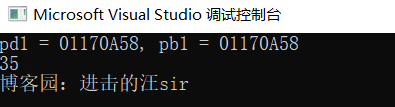pd 2指向的是整型变量 n，并没有指向一个 Derived 类的对象，在使用 dynamic_cast 进行类型转换时也没有检查这一点因为向上转型始终是安全的，所以 dynamic_cast 不会进行任何运行期间的检查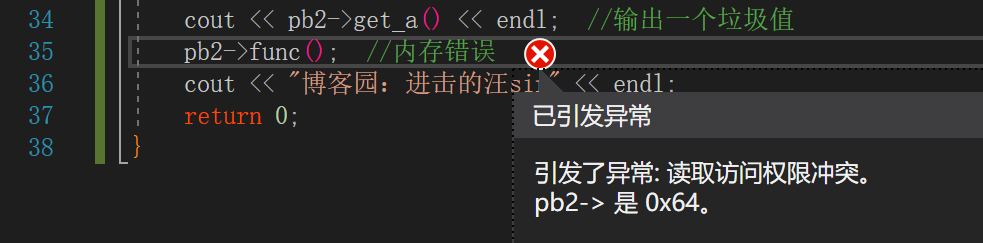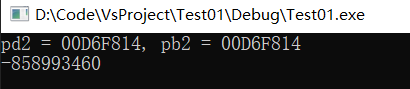### 2.3.2 向下转型（Downcasting）

#include <iostream>
using namespace std;
class A{
public:
virtual void func() const { cout<<"Class A"<<endl; }
private:
int m_a;
};
class B: public A{
public:
virtual void func() const { cout<<"Class B"<<endl; }
private:
int m_b;
};
class C: public B{
public:
virtual void func() const { cout<<"Class C"<<endl; }
private:
int m_c;
};
class D: public C{
public:
virtual void func() const { cout<<"Class D"<<endl; }
private:
int m_d;
};
int main(){
A *pa = new A();
B *pb;
C *pc;

//情况①
pb = dynamic_cast<B*>(pa);  //向下转型失败
if(pb == NULL){
cout<<"Downcasting failed: A* to B*"<<endl;
}else{
cout<<"Downcasting successfully: A* to B*"<<endl;
pb -> func();
}
pc = dynamic_cast<C*>(pa);  //向下转型失败
if(pc == NULL){
cout<<"Downcasting failed: A* to C*"<<endl;
}else{
cout<<"Downcasting successfully: A* to C*"<<endl;
pc -> func();
}

cout<<"-------------------------"<<endl;

//情况②
pa = new D();  //向上转型都是允许的
pb = dynamic_cast<B*>(pa);  //向下转型成功
if(pb == NULL){
cout<<"Downcasting failed: A* to B*"<<endl;
}else{
cout<<"Downcasting successfully: A* to B*"<<endl;
pb -> func();
}
pc = dynamic_cast<C*>(pa);  //向下转型成功
if(pc == NULL){
cout<<"Downcasting failed: A* to C*"<<endl;
}else{
cout<<"Downcasting successfully: A* to C*"<<endl;
pc -> func();
}

return 0;
}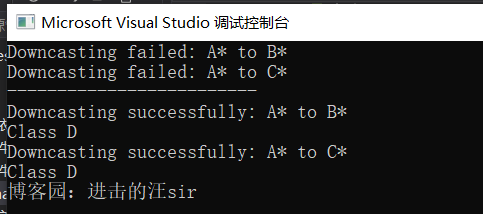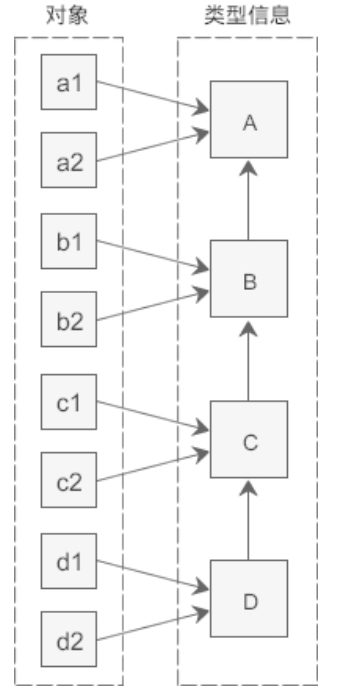# 3. 参考链接

http://c.biancheng.net/cpp/biancheng/view/3297.html

posted @ 2021-07-24 12:12  进击的汪sir  阅读(201)  评论(0编辑  收藏  举报